Home > CCA2 > Chapter 12 > Lesson 12.1.1 > Problem12-15

12-15.
1. If π < θ <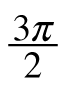, what must be true about the sign of: Homework Help ✎

1. sin(θ)

2. cos(θ)

3. tan(θ)

4.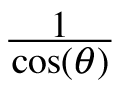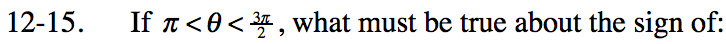Graph each function using the input bar below or on your graphing calculator. What do you observe about the y-values when θ is between π and /2?

When graphing using the input bar, sin(θ) is equivalent to sin(x).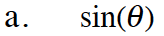The y-values are negative.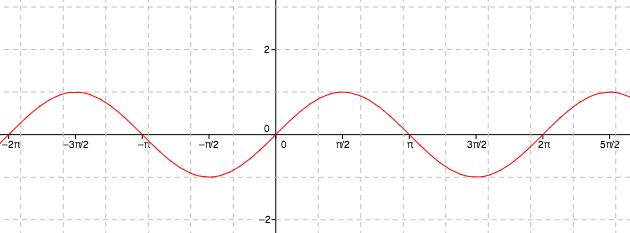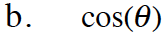Use the same method as part (a). It might also help to think about how the sin(x) and cos(x) graphs relate to each other.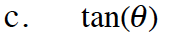Use the same method as part (a).

Negative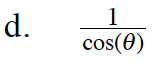Does taking the reciprocal of something change its sign?